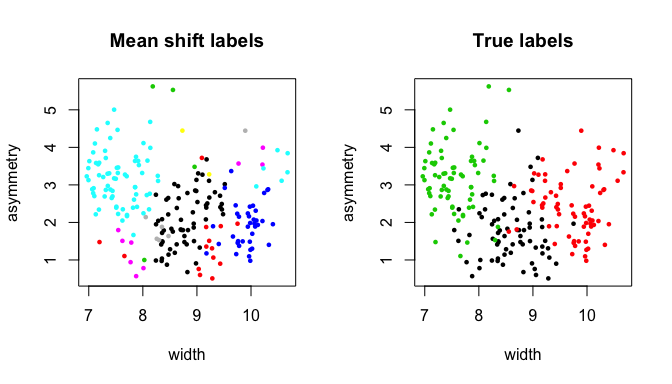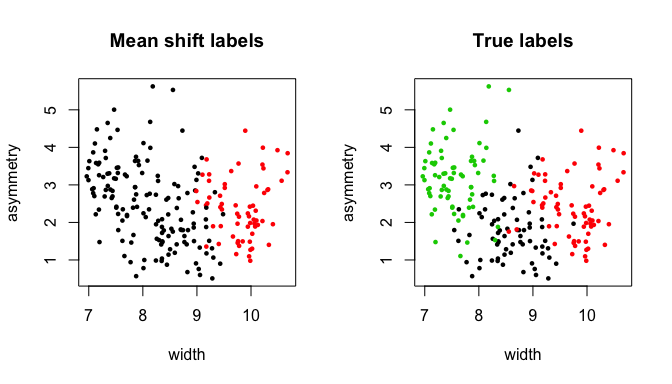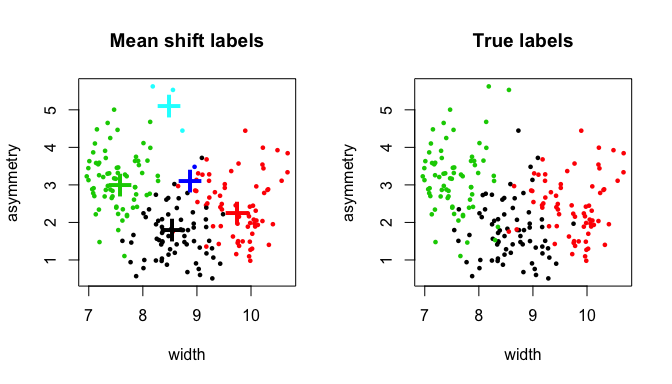## The mean-shift algorithm and modal clustering

The mean shift algorithm (Fukunaga and Hostetler 1975; Cheng 1995) is a recursive algorithm that allows us to perform nonparametric mode-based clustering, i.e. clustering data on the basis of a kernel density estimate of the probability density function associated with the data-generating process. https://normaldeviate.wordpress.com/2012/07/20/the-amazing-mean-shift-algorithm/ has a great introduction to the mean shift algorithm.

In its standard form, the mean shift algorithm works as follows. We observe $$X_1, \dots, X_n$$, a sample of i.i.d. random variables valued in $$\mathbb{R}^d$$ generated from an unknown probability density $$p$$. We fix a kernel function $$K$$ and a bandwidth parameter $$h$$ and we apply the update rule $x \leftarrow \frac{\sum_{i=1}^n K \left( \frac{\|X_i-x\|}{h} \right)X_i}{\sum_{i=1}^n K \left( \frac{\|X_i-x\|}{h} \right)}$ to an arbitrary initial point $$x=x_0 \in \mathbb{R}^d$$ until convergence. The discrete sequence of points $${x_0, x_1, \dots, x_k, \dots}$$ generated by the application of the above rule approximates the continuous gradient flow trajectory $$\pi_x$$ satisfying $\begin{cases} \pi_x(0)=x_0\\ \pi_x'(t)=\nabla \hat p(\pi_x(t)) \end{cases}$ where $$\hat p$$ is a kernel density estimator of $$p$$ based on another kernel function (the “shadow” kernel of $$K$$). In turn, $$\pi_x$$ is an estimate of the population gradient flow line $$\tau_x$$ satisfying $\begin{cases} \tau_x(0)=x_0\\ \tau_x'(t)=\nabla p(\tau_x(t)) \end{cases}$ associated to the population gradient flow based on $$p$$. Under some assumptions on $$p$$ and $$K$$, it can be shown that $$\pi_x(t)$$ and $$\tau_x(t)$$ converge respectively to a mode (a local maximum) of $$\hat p$$ and $$p$$ as $$t \to \infty$$. Furthermore, for any initial point $$x \in \mathbb{R}^d$$, there is a unique $$\pi_x$$ and a unique $$\tau_x$$, and the collections $$\{\tau_x\}_{\{x \in \mathbb{R}^d\}}$$ and $$\{\pi_x\}_{\{x \in \mathbb{R}^d\}}$$ both partition $$\mathbb{R}^d$$, thus inducing respectively a population and an empirical clustering. More specifically, a set $$M$$ in the population partition (or “population clustering”) induced by $$\{\tau_x\}_{\{x \in \mathbb{R}^d\}}$$ can be described as the subset of points in $$\mathbb{R}^d$$ such that $$\tau_x(t) \to m$$ as $$t \to \infty$$, where $$m$$ is a mode of $$p$$, i.e. $$M=\{x \in \mathbb{R}^d: \lim_{t \to \infty} \tau_x(t) = m \}$$. In a similar way, $$\hat M=\{x \in \mathbb{R}^d: \lim_{t \to \infty} \pi_x(t) = \hat m \}$$ defines an “empirical cluster”. For more details, see for instance Arias-Castro, Mason, and Pelletier (2013) and Chacón (2015).

From a practical point of view, it is clear that one is particularly interested in the case $$x=x_0 \in \{X_1,\dots,X_n\}$$ as we want to group the sample data into “sample clusters”. The MeanShift package is designed to accomplish this goal.

## The “MeanShift” package

The MeanShift package contains two implementations of the mean shift algorithm: the standard mean shift algorithm and its “blurring” version, which is an approximation to the standard algorithm that is often substantially faster.

The standard implementation of the mean shift algorithm comes with the function msClustering. The user needs to input

• X: the data matrix containing the sample points $$\{X_1, \dots, X_n\}$$ by column.

• h: the bandwidth parameter.

• kernel: the type of kernel function $$K$$

• tol.stop: a tolerance parameter; the mean shift update equation is stopped at iteration $$k$$ if $$\|x_k-x_{k-1}\|<$$tol.stop.

• tol.epsilon: another tolerance parameter; once the mean shift algorithm has been applied to all the columns of X, the $$X_i$$ is assigned to the cluster corresponding to the mode $$\hat m$$ if the end point of its mean shift trajectory lies within tol.epsilon from $$\hat m$$. These assignments are implemented using an efficient algorithm to identify connected components. See Carreira-Perpinán (2015) for more details.

• multi.core: a logical parameter that allows to parallelize the algorithm using multiple cores.

In our implementation, convergence is achieved at iteration $$k$$ if $$\|x_k - x_{k-1}\|<$$tol.stop.

The blurring mean shift algorithm is a variant of the mean shift algorithm in which the sample $$\{X_1,\dots,X_n\}$$ is updated at each mean shift iteration. In particular, $$\forall i \in {1,\dots,n}$$, the update $X_i \leftarrow \frac{\sum_{j=1}^n K \left( \frac{\|X_j-X_i\|}{h} \right)X_j}{\sum_{j=1}^n K \left( \frac{\|X_j-X_i\|}{h} \right)}$ is recursively applied until convergence. In the MeanShift package, the blurring mean shift algorithm is available with the function bmsClustering which takes the following input arguments:

• X, h, kernel, tol.stop, tol.epsilon: same as in msClustering.

• max.iter: a maximum number of iterations; if convergence does not occur in max.iter iterations, the algorithm is interrupted.

In the context of bmsClustering, convergence occurs at the $$k$$-th iteration if $$\max_i \|X_{i,k}-X_{i,k-1}\|<$$ tol.stop.

## Example: clustering wheat grain varieties

We illustrate the use of the MeanShift package by applying it to the seeds dataset at https://archive.ics.uci.edu/ml/datasets/seeds. The seeds dataset gives measurements of geometrical properties of wheat grains belonging to 3 different varieties.

Our goal is to demonstrate the use of the msClustering and bmsClustering functions by clustering the wheat varieties on the basis of the 7 quantitative variables contained in the dataset.

## load "MeanShift" package
library( MeanShift )

## load seeds dataset
load( "seeds.RData" )
## wheat variety labels
seeds.labels <- seeds[,"variety"]

## organize data by columns
seeds.data <- t( seeds[,c( "area", "perimeter", "compactness",
"length", "width", "asymmetry",
"groove.length" )] )

print( dim( seeds.data ) )
##    7 210
## standardize the variables
seeds.data <- seeds.data / apply( seeds.data, 1, sd )

## form a set of candidate bandwidths
h.cand <- quantile( dist( t( seeds.data ) ), seq( 0.05, 0.40, by=0.05 ) )
## perform mean shift clustering with the blurring version of the algorithm
system.time( bms.clustering <- lapply( h.cand,
function( h ){ bmsClustering( seeds.data, h=h ) } ) )
##    user  system elapsed
##  14.797   0.243  15.075
## the resulting object is a list with names "components" and "labels"
class( bms.clustering[] )
##  "list"
names( bms.clustering[] )
##  "components" "labels"
## extract the cluster labels
ms.labels1 <- bms.clustering[]$labels print( ms.labels1 ) ##  1 1 1 1 2 1 1 1 2 2 1 1 1 1 1 1 3 2 4 5 1 1 2 ##  6 1 2 5 5 1 5 6 1 1 1 1 2 2 2 1 7 1 1 8 9 1 1 ##  1 1 1 1 1 10 1 1 1 1 1 2 1 11 6 6 8 5 6 8 1 1 1 ##  5 9 9 9 12 9 9 9 13 12 2 9 14 13 12 12 12 12 12 13 13 12 12 ##  12 14 15 9 12 12 12 12 9 12 12 12 12 12 12 9 12 12 12 12 12 16 13 ##  12 12 12 12 12 13 9 9 12 1 12 12 12 12 14 12 12 9 9 9 1 9 17 ##  17 9 5 5 5 5 5 5 18 5 5 5 5 5 5 5 5 5 5 5 5 5 5 ##  5 5 5 5 6 5 5 5 5 5 5 5 5 5 5 5 5 5 18 5 5 5 5 ##  5 5 5 5 5 5 5 5 5 5 5 5 5 5 5 8 5 8 5 19 5 5 5 ##  19 5 5 ## extract the cluster modes/representatives ms.modes1 <- bms.clustering[]$components
print( ms.modes1 )
##                   mode1     mode2     mode3     mode4     mode5     mode6
## area           4.972157  5.457998  4.808057  4.948616  4.088405  4.202809
## perimeter     11.009962 11.462657 10.589921 10.816368 10.156949 10.151372
## compactness   37.205021 37.664099 38.862576 38.381917 35.921579 36.956050
## length        12.525640 12.899283 11.553649 11.871491 11.820522 11.624340
## width          8.615213  9.155608  8.956502  8.976540  7.545743  7.819524
## asymmetry      1.713081  1.293471  3.481078  1.432936  3.064897  1.244180
## groove.length 10.449043 10.855776  9.727751  9.684061 10.390169  9.928533
##                   mode7     mode8     mode9    mode10     mode11    mode12
## area           4.907723  4.443284  5.770750  5.423241  4.1619419  6.501662
## perimeter     10.850266 10.315128 11.901281 11.416900 10.3142616 12.521584
## compactness   37.851125 37.878503 36.943143 37.762253 35.5150537 37.620858
## length        12.181099 11.540933 13.471424 12.806291 11.6439297 14.041477
## width          8.731464  8.351152  9.206832  9.091524  8.0272281  9.950198
## asymmetry      4.446123  1.743388  2.811806  3.719845  0.9989644  1.915542
## groove.length 10.175378  9.650353 11.916932 10.450059  9.1946680 12.382798
##                  mode13    mode14    mode15    mode16    mode17    mode18
## area           7.099928  6.381724  6.309930  6.577999  5.362699  4.015062
## perimeter     13.067127 12.322257 12.649711 12.718626 11.584104 10.126733
## compactness   37.728747 38.122775 35.768975 36.911618 36.250626 35.503521
## length        14.562598 13.576357 15.045248 14.126644 13.247384 11.832339
## width         10.471816 10.119505  9.226547  9.893717  8.652357  7.380860
## asymmetry      3.423494  3.244469  3.280886  4.444128  1.776517  1.972903
## groove.length 12.762805 11.933930 13.119544 12.315850 11.783123 10.411936
##                  mode19
## area           4.450632
## perimeter     10.364034
## compactness   37.573928
## length        11.757909
## width          8.370080
## asymmetry      5.577108
## groove.length 10.230314
## plot
par( mfrow=c( 1, 2 ) )
plot( seeds.data[5,], seeds.data[6,], col=bms.clustering[]$labels, xlab=names( seeds ), ylab=names( seeds ), main="Mean shift labels", cex=0.65, pch=16 ) plot( seeds.data[5,], seeds.data[6,], col=seeds.labels, xlab=names( seeds ), ylab=names( seeds ), main="True labels", cex=0.65, pch=16 )## bandwidth h is too small -> "overclustering" ## extract the cluster labels ms.labels6 <- bms.clustering[]$labels
print( ms.labels6 )
##    1 1 1 1 1 1 1 1 2 1 1 1 1 1 1 1 1 1 1 1 1 1 1 1 1 1 1 1 1 1 1 1 1 1 1
##   1 1 2 1 1 1 1 1 1 1 1 1 1 1 1 1 1 1 1 1 1 1 1 1 1 1 1 1 1 1 1 1 1 1 1
##   2 2 2 2 2 2 2 2 2 2 2 2 2 2 2 2 2 2 2 2 2 2 2 2 2 2 2 2 2 2 2 2 2 2 2
##  2 2 2 2 2 2 2 2 2 2 2 2 2 2 2 2 2 2 2 1 2 2 2 2 2 2 2 1 2 2 1 2 1 1 2
##  1 1 1 1 1 1 1 1 1 1 1 1 1 1 1 1 1 1 1 1 1 1 1 1 1 1 1 1 1 1 1 1 1 1 1
##  1 1 1 1 1 1 1 1 1 1 1 1 1 1 1 1 1 1 1 1 1 1 1 1 1 1 1 1 1 1 1 1 1 1 1
## extract the cluster modes/representatives
ms.modes6 <- bms.clustering[]$components print( ms.modes6 ) ## mode1 mode2 ## area 4.537369 6.230689 ## perimeter 10.582507 12.278929 ## compactness 36.594028 37.413398 ## length 12.154444 13.809121 ## width 8.093357 9.684194 ## asymmetry 2.458180 2.271504 ## groove.length 10.445110 12.123523 ## plot par( mfrow=c( 1, 2 ) ) plot( seeds.data[5,], seeds.data[6,], col=bms.clustering[]$labels,
xlab=names( seeds ), ylab=names( seeds ), main="Mean shift labels",
cex=0.65, pch=16 )
plot( seeds.data[5,], seeds.data[6,], col=seeds.labels,
xlab=names( seeds ), ylab=names( seeds ), main="True labels",
cex=0.65, pch=16 )## bandwidth h is too large -> "underclustering"

## extract the cluster labels
ms.labels3 <- bms.clustering[]$labels print( ms.labels3 ) ##  1 1 1 1 1 1 1 1 2 1 1 1 1 1 1 1 4 1 1 3 1 1 1 1 1 1 1 1 1 1 1 1 1 1 1 ##  1 1 2 1 5 1 1 1 2 1 1 1 1 1 1 1 1 1 1 1 1 1 1 1 1 3 1 1 3 1 1 1 1 1 3 ##  2 2 2 2 2 2 2 2 2 2 2 2 2 2 2 2 2 2 2 2 2 2 2 2 2 2 2 2 2 2 2 2 2 2 2 ##  2 2 2 2 2 2 2 2 2 2 2 2 2 2 2 2 2 2 2 1 2 2 2 2 2 2 2 2 2 2 1 2 2 1 2 ##  3 3 3 3 3 3 3 3 3 3 3 3 3 3 3 3 3 3 3 3 3 3 3 3 3 1 3 3 3 3 3 3 3 3 3 ##  3 3 3 3 3 3 3 3 3 3 3 3 3 3 3 3 3 3 3 3 3 3 3 3 1 3 1 3 5 3 3 3 5 3 3 ## extract the cluster modes/representatives ms.modes3 <- bms.clustering[]$components
print( ms.modes3 )
##                   mode1     mode2     mode3     mode4     mode5
## area           4.892242  6.317891  4.118544  4.873240  4.618885
## perimeter     10.915456 12.365544 10.187833 10.718496 10.547214
## compactness   37.182117 37.436694 35.951363 38.468425 37.626968
## length        12.408678 13.896625 11.846968 11.791901 11.930316
## width          8.544545  9.759001  7.578098  8.881919  8.495775
## asymmetry      1.812428  2.249885  2.997756  3.127102  5.111517
## groove.length 10.424372 12.237327 10.390129  9.797439 10.246984
## plot
par( mfrow=c( 1, 2 ) )
plot( seeds.data[5,], seeds.data[6,], col=bms.clustering[]\$labels,
xlab=names( seeds ), ylab=names( seeds ), main="Mean shift labels",
cex=0.65, pch=16 )
## add estimated cluster modes to the plot
points( ms.modes3[5,], ms.modes3[6,], col=1:ncol( ms.modes3 ),
pch="+", cex=3 )
plot( seeds.data[5,], seeds.data[6,], col=seeds.labels,
xlab=names( seeds ), ylab=names( seeds ), main="True labels",
cex=0.65, pch=16 )## just right!

Arias-Castro, Ery, David Mason, and Bruno Pelletier. 2013. “On the Estimation of the Gradient Lines of a Density and the Consistency of the Mean-Shift Algorithm.” Unpublished Manuscript.

Carreira-Perpinán, Miguel A. 2015. “A Review of Mean-Shift Algorithms for Clustering.” ArXiv Preprint ArXiv:1503.00687.

Chacón, José E. 2015. “A Population Background for Nonparametric Density-Based Clustering.” Statistical Science 30 (4): 518–32.

Cheng, Yizong. 1995. “Mean Shift, Mode Seeking, and Clustering.” IEEE Transactions on Pattern Analysis and Machine Intelligence 17 (8): 790–99.

Fukunaga, Keinosuke, and Larry Hostetler. 1975. “The Estimation of the Gradient of a Density Function, with Applications in Pattern Recognition.” IEEE Transactions on Information Theory 21 (1): 32–40.